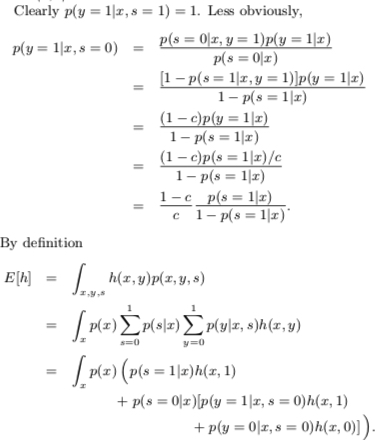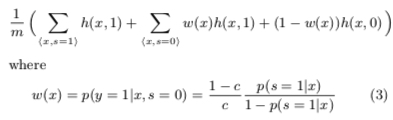1. CVR 模型的训练
2. 基于后验的调价策略

## Modeling Delayed Feedback in Display Advertising(2014)

《Modeling Delayed Feedback in Display Advertising》 阅读笔记

## A Nonparametric Delayed Feedback Model for Conversion Rate Prediction(2018)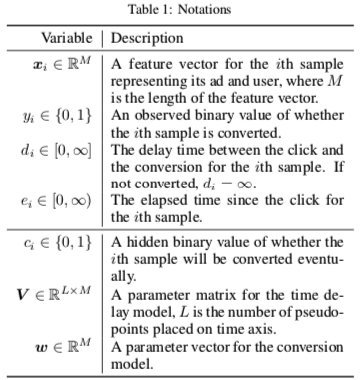Paper 中主要通过 Survival analysis 的方法来推导, 简单来说，survival analysis 主要研究如下领域

Survival analysis is a branch of statistics for analyzing the expected duration of time until one or more events happen, such as death in biological organisms and failure in mechanical systems.

Paper 中使用到的 survival analysis 概念和推论如下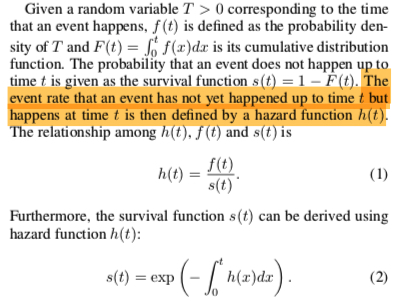$$h(d_i; x_i, V) = \sum_{l=1}^{L}\alpha_l(x_i;V)k(t_l, d_i)$$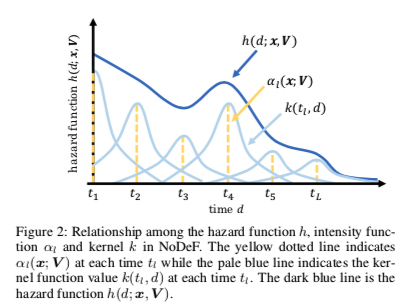$$\alpha_l(x_i; V) = (1+\exp(-V_{l}^{T}x_i))^{-1}$$

$$k(t_l, \tau) = \exp(-\frac{(t_l-\tau)^2}{2h^2})$$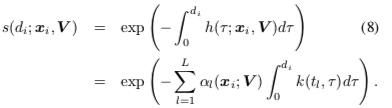$$p(d_i|x_i, c_i = 1) = s(d_i; x_i, V )h(d_i; x_i, V)$$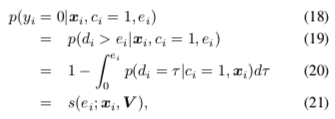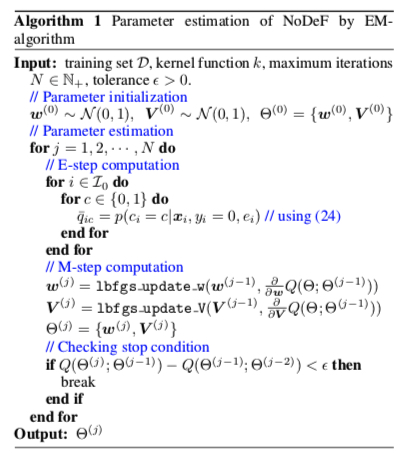## Addressing Delayed Feedback for Continuous Training with Neural Networks in CTR prediction(2019)

### Faked nagative weighted

Delay feedback 的问题也可以从另一个角度去理解，观察到的样本的分布是 biased distribution $b$,但是需要求解真实的样本的分布 $p$的期望；而 importance sampling 是解决这个问题的一个方法，这个方法在 Wikipedia 上的简单介绍如下

In statistics, importance sampling is a general technique for estimating properties of a particular distribution, while only having samples generated from a different distribution than the distribution of interest

zhihu 上一个更通俗的解释见 重要性采样（Importance Sampling），则 paper 中的可将真实分布的期望写成如下形式

$$E_p[\log f_{\theta}(y|x)] = E_b[\frac{p(x,y)}{b(x,y)} f_{\theta}(y|x)]$$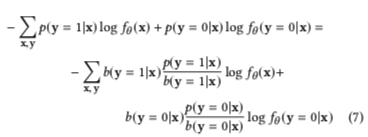$$b(y=1|x) = \frac{M}{M+N} = \frac{\frac{M}{N}}{1+\frac{M}{N}} = \frac{p(y=1|x)}{1+p(y=1|x)}$$

$$b(y=0|x) = 1- b(y=1|x)= \frac{1}{1+p(y=1|x)}$$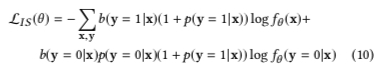### Fake negative calibration

$$b(y=1|x) = \frac{M}{M+N} = \frac{\frac{M}{N}}{1+\frac{M}{N}} = \frac{p(y=1|x)}{1+p(y=1|x)}$$

$$p(y=1|x) = \frac{b(y=1|x)}{1-b(y=1|x)}$$

$$\frac{P}{P+N} = \frac{1}{1+e^{-x}}$$

$$\frac{P}{r*(P+N)+P} = \frac{1}{1+e^{-y}}$$

$$\frac{P}{r*(P+N)+P} = \frac{P/(P+N)}{r+P/(P+N)} = \frac{1/(1+e^{-x})}{r + 1/(1+e^{-x})}$$

$$\frac{1/(1+e^{-x})}{r + 1/(1+e^{-x})}=\frac{1}{1+e^{-y}}$$

$$y = -(\ln r+ \ln(1+e^{-x}))$$

### Positive-Unlabeled Learning

paper 关于里面基本没有推导，详细的推导可参考 Learning Classifiers from Only Positive and Unlabeled Data, 基本思路跟第一篇比较像，这里就不详细展开了，主要引用了里面一些关键的推导步骤

A key assumption about the training data is that they are drawn randomly from $p(x,y,s)$, and for each tuple $< x,y,s >$ that is drawn, only $< x,s >$ is recorded. Here $s$ is the observed label and $y$ is the actual label, which might not have occurred yet. Along with this, it is assumed that labeled positive examples are chosen completely randomly from all positive examples, i.e. $p(s = 1|x, y = 1) = p(s = 1|y = 1)$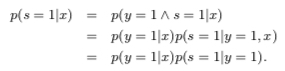The value of the constant $c = p(s = 1|y = 1)$ can be estimated using a trained classifier $g$ and a validation set of examples. Let $V$ be such a validation set that is drawn from the overall distribution $p(x, y, s)$ in the same manner as the nontraditional training set. Let $P$ be the subset of examples in $V$ that are labeled (and hence positive). The estimator of $p(s = 1|y = 1)$ is the average value of $g(x)$ for $x$ in $P$. That is $\frac{1}{n}\sum_{x \in P}g(x)$